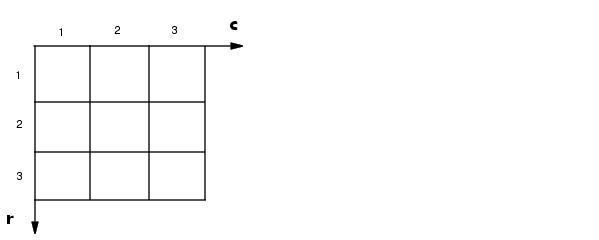Image Processing Toolbox User's GuidePixel Coordinates

Generally, the most convenient method for expressing locations in an image is to use pixel coordinates. In this coordinate system, the image is treated as a grid of discrete elements, ordered from top to bottom and left to right, as illustrated by the following figure.The Pixel Coordinate System

For pixel coordinates, the first component `r` (the row) increases downward, while the second component `c` (the column) increases to the right. Pixel coordinates are integer values and range between 1 and the length of the row or column.

There is a one-to-one correspondence between pixel coordinates and the coordinates MATLAB uses for matrix subscripting. This correspondence makes the relationship between an image's data matrix and the way the image is displayed easy to understand. For example, the data for the pixel in the fifth row, second column is stored in the matrix element (5,2).Coordinate Systems Spatial Coordinates